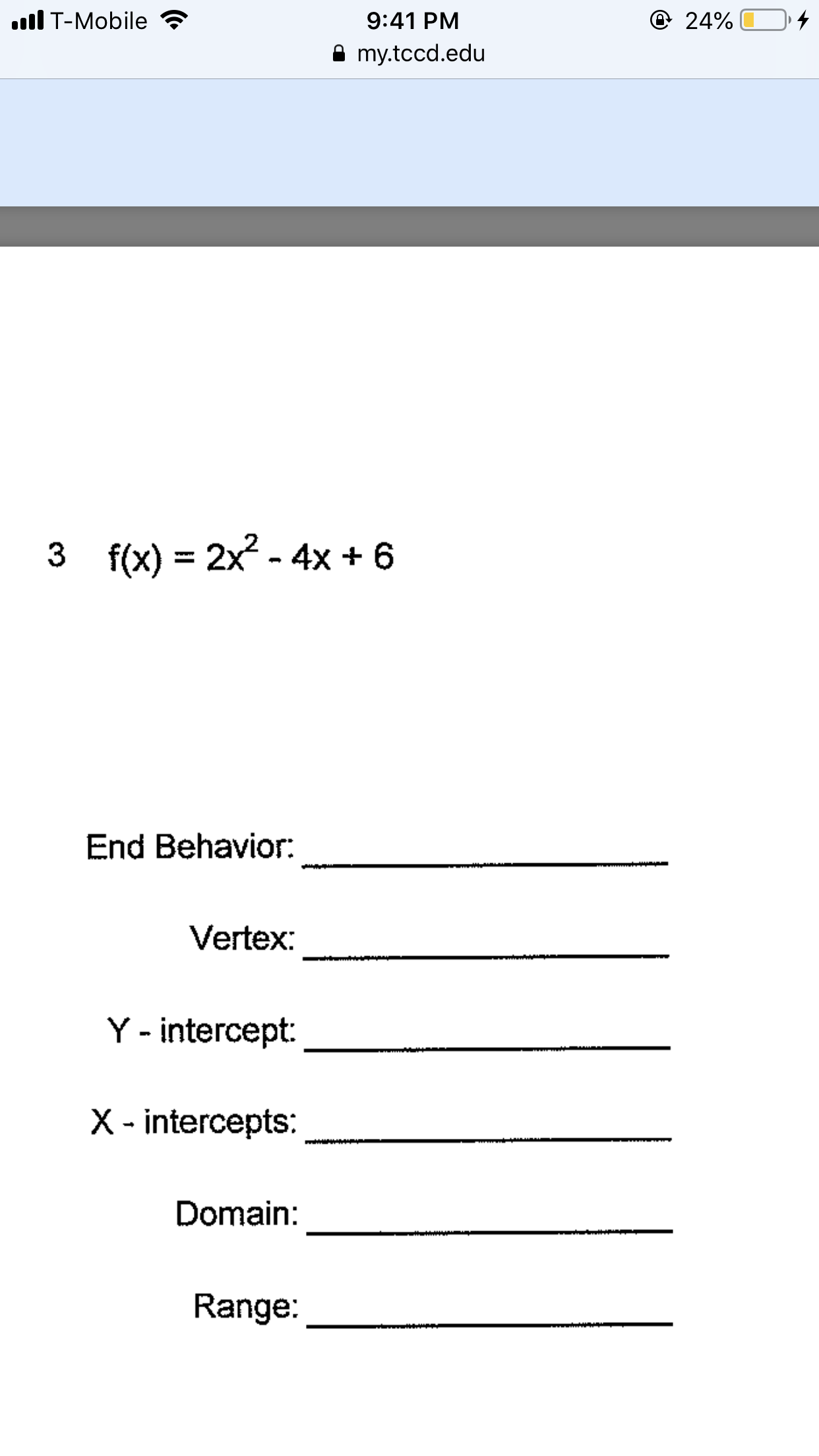# lT-Mobile9:41 PM24% D.my.tccd.edu3f(x) - 2x6- 4x +End Behavior.Vertex:Y - intercept:X -intercepts:Domain:Range:

Question
4 views

Help solving ithelp_outlineImage TranscriptioncloselT-Mobile 9:41 PM 24% D. my.tccd.edu 3 f(x) - 2x6 - 4x + End Behavior. Vertex: Y - intercept: X -intercepts: Domain: Range: fullscreen
check_circle

Step 1
• End Behavior:

As the leading term, it means the polynomial which has the highest power of the variable is 2x2, which is an even term, it will always have the positive values for any value of x.

It means that f(x)→∞ as x→ -∞ and f(x)→∞ as x→∞

The graph of the function is shown on the board:

Step 2
• Vertex:

The function is the form of parabola, according its definition it is given as:

y=ax2+bx+c, its vertex is given as x=-b/2a

As the given function is f(x)= 2x2-4x+6,

here a=2, b=-4, c=6

therefore, vertex calculation is shown on the board:

Therefore, the vertex is given for the function is (1,4).

Step 3
• Y-intercept:

y-intercept is a point on the graph where x=0

f(x)= 2x2-4x+6 when x=0

f(x) = 6

So, its y- intercept is (0,6).

• X-intercepts:

x-intercept is a point on the graph where y=0

To determine its intercept we calculate its discriminant, for polynomial function its discriminant is calculated as b2-4ac.

Here, a=2, b=-4, c=6

D= (-4)2-4 X 2 X 6=-32

Discriminant cannot be negative for x € R...

### Want to see the full answer?

See Solution

#### Want to see this answer and more?

Solutions are written by subject experts who are available 24/7. Questions are typically answered within 1 hour.*

See Solution
*Response times may vary by subject and question.
Tagged in

### Applications of Mathematics